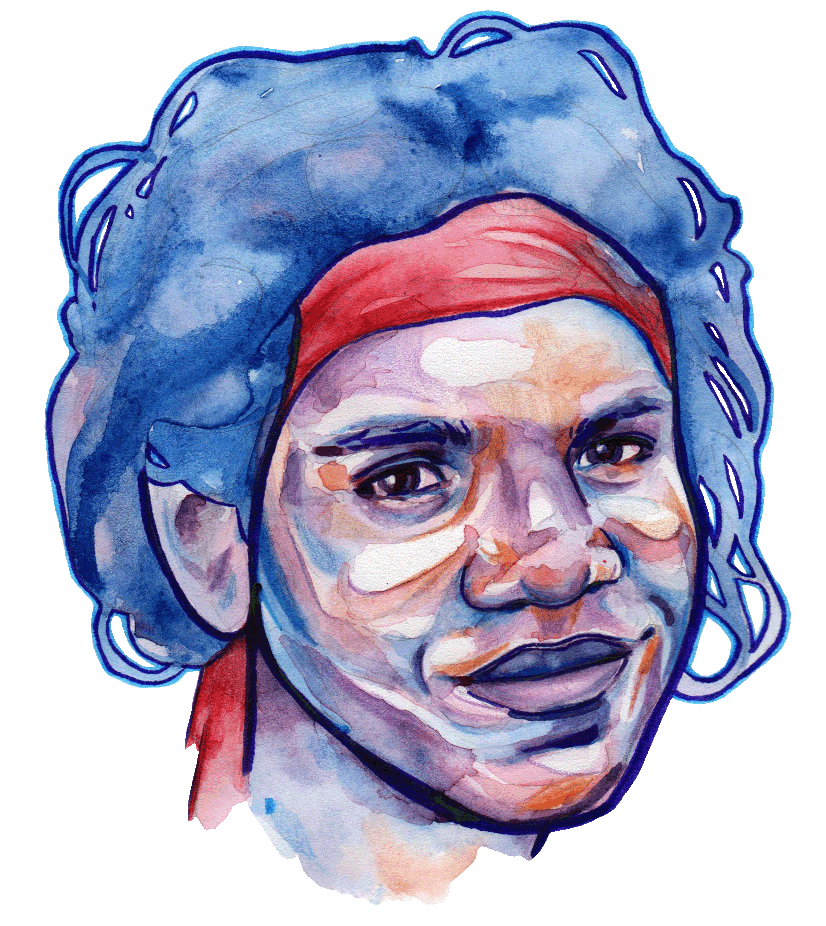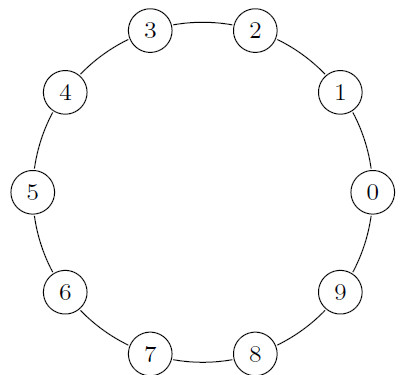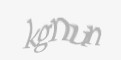Let me introduce you SCA. SCA is an Aboriginal cryptographer living in the Australian bush. She has never learned to read or to do arithmetic.One day, SCA finds some sheets of paper. On the first one, one could read:

 383+386=769;277+415=692;293+335=628;386+492=878;149+421=570;362+27=389;190+59=249;263+426=689;40+426=466;172+236=408;211+368=579;334-193=141;439-334=105;421-159=262;485-457=28;354-261=93;472-262=210;216-41=175;352-350=2;482-162=320;399-217=182;368x42=15456;247x0=0;155x462=71610;436x131=57116;71x217=15407;458x138=63204;476x187=89012;17x434=7378;199x140=27860;270x72=19440;

SCA finds 50 sheets of mathematical sentences like this one and gets very excited. What could she learn from them? She does not know what ciphers are. They represent for her merely various incomprehensible symbols. How could she possibly tell that the symbol 8 on the sheet represents the number eight?

Let me tell you how SCA learns, in only four steps, the decimal system and how to do arithmetic by applying a semiotic algorithm. She follows the algorithm using a stick to draw lines on the sand and piling together stones to keep count.

# Step 1

SCA discovers that there are 15 different symbols on the sheets (the ciphers, the signs of addition, subtraction and equality and the semicolon).

She counts their total occurrences and the occurrences of any sequence of two symbols. Certain symbols never appear in a sequence next to each other: they are the symbols belonging to the group [;+-x=].

All other symbols form another group or paradigm: .

# Step 2

SCA discovers a pattern having sequences of symbols in the paradigm [0...9] separated by one symbol = and then by one symbol ; and then by one symbol that could be either + or - or x.

SCA looks for more specific patterns. For example, at the beginning of the first sheet, a sub-pattern having both a symbol 7 following the symbol = and a symbol 2 following the symbol ; is observed. SCA knows the number of occurrences of the sequence of symbols = and 7, and of the sequence of symbols ; and 2. A priory, she would expect the sub-pattern to occur a number of times equal to the ratio of the product of the occurrences of the two sequences over of the number of occurrences of the symbol =. Empirically, SCA counts the number of occurrences of this sub-pattern, to compare it with the expected number of occurrences. It occurs 17 times while she would have expected it 22 times. SCA decides that this sub-pattern is not relevant. SCA repeats for all sub-patterns this operation of comparing the expected number of occurrences and the empirical number of occurrences. Relevant sub-patterns she finds include:

• the sub-pattern having both a symbol 7 preceding the symbol + and a symbol 8 preceding the symbol = and a symbol 5 preceding the symbol ;
• the sub-pattern having both a symbol 7 preceding with a given offset  the symbol + and a symbol 8 preceding with the same offset the symbol = and a symbol 5 preceding with the same offset the symbol ;
• the sub-pattern having both a symbol 7 preceding with a given offset  the symbol + and a symbol 8 preceding with the same offset the symbol = and a symbol 6 preceding with the same offset the symbol ; (like in ...79+382=561;...)

Not all sub-patterns having this structure are retrieved by SCA from the sheets and also sub-patterns having different structures are retrieved. When two sub-patterns differing only by one symbol are retrieved, SCA deduces that the distinguishing symbols must be related (in the example, the symbols 5 and 6).

SCA forms the paradigms , , , , , , and so on. She is surprised to find out that the new paradigms form a chain.A puzzling observation is made by SCA. Symbols which are adjacent in the chain graph appear to be related to the symbol 1 in observations like ...;149+421=570;... or ...;354-261=93;... or ...;472-262=210;...

# Step 3

SCA creates original paradigms from the chain graph of step 2: the paradigm of two-hop neighbors (02|13|24|35|46|57|68|79|80|91), the paradigm of three-hop neighbors (03|14|25|36|47|58|69|70|81|92), and so on.

Symbols in these paradigms appear to be related to the symbols 2 and + (or the symbols 2 and -), 3 and + (or the symbols 3 and -), and so on.

SCA realizes that the symbols in the paradigm [0...9] indicate numerosities. She grounds the originally incomprehensible symbol 1 as a representation of the numerosity of one and she does the same for all the other ciphers (she knows only how to represent numbers up to nine). She also grounds the complementarity of addition and subtraction.

In step 3, SCA also discovers the paradigm of pairs of ciphers summing more than ten and the paradigm of ordered pairs of ciphers, which she can use to solve, respectively, multi-cipher additions and subtractions.

# Step 4

SCA makes the hypothesis that adding two ciphers means, starting from the first cipher,  taking a number of hops given by the other one. Adding ciphers summing more than ten involves stepping once through the cipher 0. Similarly, subtracting from a first cipher a greater one involves stepping through the cipher 0. SCA discovers that the number of passages through zero is the carryover and grounds the properties of the place-value representation.

Finally, SCA understands that multiplication involves repeated addition, which involves one or more passages through zero. She then learns how to solve multiplication.

# From SCA to the Semiotic Cognitive Automaton

The semiotic algorithm, used by the Aboriginal cryptographer to learn the Arabic decimal system and how to do arithmetic, can be programmed into a machine to obtain a Semiotic Cognitive Automaton.

Like the Aboriginal, the automaton discovers, when provided with a corpus of mathematical sentences:

• in step 1, a paradigm of symbols corresponding to the ciphers;
• in step 2, an ordering of the symbols in this paradigm and syntagmatic relationships of any two adjacent symbols together with the symbols + and 1 (or the symbols - and 1);
• in step 3, syntactic rules for solving multi-cipher additions and subtractions.

To be able to say that the automaton grasps semantics (cognitive grounding), it needs to link the successor function and the addition call in its program with the syntactic rules for, respectively, computing the successor and solving addition it discovers from the corpus (second-order reasoning).

One could imagine that the program run by the Semiotic Cognitive Automaton originally makes calls to functions operating in Peano arithmetic (i.e. constructing addition from repeated counting and multiplication from repeated addition). The automaton could then improve itself (self-improving Artificial Intelligence) if it replaces said function calls in its program with the rules operating in the Arabic decimal system learned by applying the semiotic algorithm to the corpus.

The original article Learning the Semantics of Notational Systems with a Semiotic Cognitive Automaton can be found here or on Springer Link.